# LOG#063. SM(V): Gauge fixing.Gauge theories require that we select “a gauge” in order to calculate physical observables. That is, you have to fix the gauge to eliminate field configurations that are physically equivalent ( they can not be distintinguished, as field configurations).

The gauge fixing procedure is very hard of practically impossible for non-abelian YM theories unless you work with the so-called “functional approach”, isung some devices invented by Feynman himself and called path integrals ( you can imagine path integrals as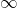-iterated integrals, or infinite differential forms somehow but we will not require a high understanding of these topics, since my blog posts on the SM basics don’t pretend to cover such advanced topics in a very precise formulation, but if you are interested, learn more about path integrals).

Using the path integral or functional approach, theoretical physicists have to apply a technique, called Fadeev-Popov (FP) method/procedure, to erase every physically equivalent field configuration in the path integral after the selection of gauge. That is, in summary, the key idea is:Moreover, the gauge fixing consists generally in a prescription of “picking some constraining functions”. Those functions can be any functions of the fields, and thus, we can distinguish two types of gauge fixing: lineal gauge fixing and non-linear gauge fixing.

The essence of the FP procedure is to restrict and constrain the functional integral/path integral: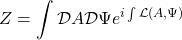The restriction is realized by a gauge-fixing condition, and it can take the form of a functional delta function: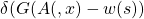and then, we perform the integration over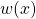with the aid of a “Gaussian” weight function. The function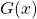is arbitrary. The method works nicely: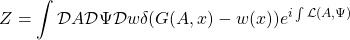and then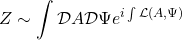## Linear gauge fixing

Linear gauge fixing is by definition a gauge fixing procedure that uses LINEAR functions in the fields, e.g.,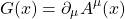Functional methods allow us to introduce two point correlation fucntions from the effective lagrangian:The gauge propagator is defined to be: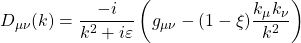This one parameter class of gauge elections is known as thegauge. Several concrete gauges have their own names in the literature due to their uses. Thus, we have 3 specially useful gauges:

1st. Landau gauge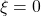.

2nd. Feynman-‘t Hooft gauge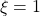.

3rd. Unitary gauge.

## Gauge fixing in the SM

In the context of SSB theories, the SM in particular, thegauge is introduced using the gauge-fixing functions defined asAfter a functional integration, the so-called gauge-fixing Lagrangian pieces arise in the SM: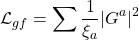and where the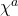fields are the Goldstone bosons corresponding to the broken gauge symmetries we select, and where the sum symbol is taken to add lagrangian pieces which are invariant under the UNBROKEN gauge symmetry of the theory left after the SSB. This choice is associated to the massive gauge boson propagators:Thus, the effect of giving mass terms for the Goldstone bosons (“modes”) are two:

1st. Goldstone bosons are proportional to the gauge parameters.

2nd. The introduction of unphysical degrees of freedom (the Goldstone modes) that are visible or existing as virtual particles inside Feynman diagrams. Something similar happens with the some extra fields we will require later, the Fadeev-Popov ghosts, for consistency. The FP ghosts will be unphysical as well. If some Goldstone massive mode survive, it is a hint of the SSB, as we will explain in a forthcoming comment.

## The unitary gauge

There are several gauge choices, as we remarked above. One of them, the unitary gauge, is particularly interesting since it erases the Goldsone boson terms completely, and then, it results in a propagator: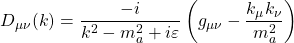for the gauge bosons. It can be obtained by sending to the infinity thein thegauge. This gauge is a very simple choice, and we don’t have to include Goldstone bosons in the perturbative calculations via Feynman diagrams. It reduces the complexity of the mathematical expressions arising in our QFT/SM. However, there is a subtle problem with this gauge. The gauge propagator does NOT fall off for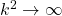and it produces some problems in the high energy limit, as an asymptotic series, specially serious if we consider loop diagrams (higher order selfinteractions, vacuum polarization effects, etc). This problem is specific to this gauge and it does not arise in thegauge. Indeed, thegauge can even be used to prove the renormalizability of general gauge theories in a general setting.

## Non linear gauge-fixing

The general FP procedure states that theconstraining functions can be arbitrary, not necessarily linear at all. Then, we should be careful to keep the lagrangian dimension equal to four as a maximum in order to protect the renormalizability of our theory. By the addition of non-linear parts to the constraining functions we wish to get even more extra gauge-fixing parameters in the total theory, and these terms are helpful to verify the correction of our final results.

Therefore, to keep things clear and neat. The linear gauge fixing piece in the SM lagrangian is given by a term: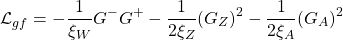and generally, most of the time and people set the gauge-fixing parameters to the same quantity:However, we can choose the constraining functions defined by: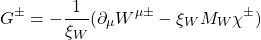We introduce additional non-linear constraining functions: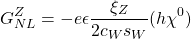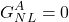Here, the Goldstone bosons are, arising after SSB,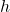is the physical Higgs boson field and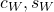are the cosine and the sine of the Weinberg angle. The parametersare new gauge fixing parameters which we can choose for free. This choice of non-linear constraints has a specaila feature: it does NOT change the quadratic part of the SM lagrangian, i.e., the propagators are NOT affected by those new gauge fixing terms, only interaction vertices ARE affected.

## Interaction vertices

If we combine the linear and non-linear gauge-fixing functions, we obtain the total gauge-fixing lagrangian piece, and it can be expanded in such a way that we can write the cubic and quartic terms in the fields, the interaction terms, explicitly! Furthermore, the quadratic parts defining the propagators of gauge fields ARE not affected by such non-linear gauge-fixing terms. In summary, the gauge-fixing of non-linear functions allow us to introduce interactions vertices in a non-trivial way that is consistent with gauge invariance! And this result is a very important theoretical fact since we did not want to spoil the gauge invariance at the end of our calculations. So, it is quite remarkable we can make all these calculations avoiding those issues.

After performing the explicit expansion of the gauge-fixing lagrangian, we can write the following seven (7 is cool number, isn’t it?) interaction vertices: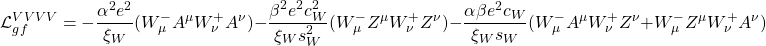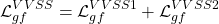with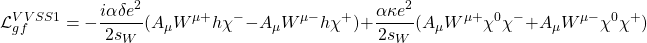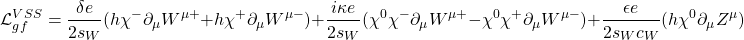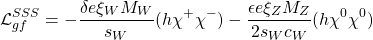In addition to these 7 interaction pieces, we have to add for inner consistency the so-called ghost field interaction terms. The ghosts are usually represented by the letter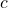and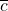. There are 5 main terms of this class in the SM: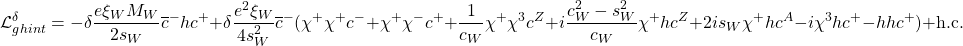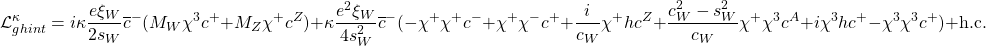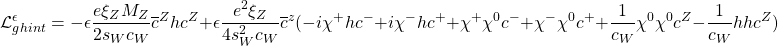## Renormalization and the SM

The whole renormalization process is a complicated procedure of any gauge theory. In the case of the SM, it can be summarized in some simple steps:

1st. Choose any set of independent physical parameters.

2nd. Separate the bare parameters and fields into two different types: renormalized parameters (fields) and renormalization constants.

3rd. Choose renormalization conditions to fix the so-called “counter-terms”.

4th. Express physical quantities as a function of the renormalized parameters.

5th. Choose input data in order to fix the value of the renormalized paramenters.

6th. Evaluate predictions for physical quantities as functions of the input data.

It sounds hard, don’t it? In fact, the first 3 conditions specify what physicists call the renormalization scheme. The most popular renormalization schemes are:

A) The on-shell scheme. It uses the knowledge that all external particles are physical, i.e, on-shell, as the boundary conditions.

B) Minimal substraction scheme (MS). It simply absosrb the divergent parts to the counterterms. More generally, in QFT,  the minimal subtraction scheme, or MS scheme, sometimes written as, is a particular renormalization scheme used to absorb the infinities that arise in perturbative calculations beyond the leading-order (tree-level). It was introduced independently by ‘t Hooft and Weinberg in 1973.  The MS scheme consists of absorbing only the divergent part of the radiative corrections into the counterterms. There is a similar and more widely used modified minimal subtraction, or, where one absorbs the divergent part plus a universal constant (which always arises along with the divergence in Feynmann diagram calculations) into the counterterms.

We are completely free to choose independent parameters in the SM. For renormalization in the Standard Model, we usually select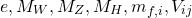Renormalization in the SM IS rather simple in principle, but it IS a very difficult and technical task. Indeed, the issue of renormalization and the mathematics behind it is even a high-tech topic for mathematical physicists and pure mathematicians. Moreover, the total landscape of renormalization in the SM is complicated by the fact that the SM includes spontaneous symmetry breaking AND flavor mixing requiring renormalization of the CKM and PMNS matrices. The set of independent parameters used as renormalization parameters in the SM is generally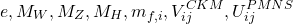Let the gauge-fixing be with you! See you in the next SM post!

This site uses Akismet to reduce spam. Learn how your comment data is processed.